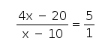# In an examination, the ratio of passes to failures was 4:1

In an examination, the ratio of passes to failures was 4:1. Had 30 less appeared and 20 less passed, the ratio of passes to failures would have been 5:1. Find the number of students who appeared for the examination.

Let the number of passed students be 4x and failed students be x.
Total number of students = 4x + x = 5x
According to the given condition,
new ratio of the number of students passed to fail students is 5: 1Solving this we gett x = 30.
Total number of students appeared in the examination is 5x = 30 × 5 = 150.